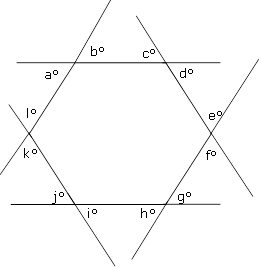# Target Test Prep GMAT Quant Challenge Problem 4

### Challenge Question

mediumIn the regular hexagon above, what is the value of a + b + c + d + e + f + g + h + i + j + k + l?

180°

360°

540°

720°

1,040°

Solution:

The sum of the exterior angles of any polygon is 360°. However, this statement is only true when we take only one exterior angle per vertex and add up only the measures of each of those angles. Here there are actually two exterior angles per vertex, a situation that will yield two sets of exterior angles. We can consider angles a, c, e, g, i, k as one set and angles b, d, f, h, j, and l as the other. Since each of these two sets of exterior angles adds up to 360°, the two sets together give us a total of 2 × 360° =  720°.